# Selina Solutions Concise Mathematics Class 6 Chapter 28 Polygons Exercise 28(B)

Selina Solutions Concise Mathematics Class 6 Chapter 28 Polygons Exercise 28(B) provides students with a clear idea of concepts which are important from the latest syllabus pattern. The solutions created by the experts are comprehensive, according to the current ICSE exam. Finding the number of sides in a regular polygon is the main concept discussed under this exercise. Frequent practice of solutions helps students to solve difficult problems within a short span of time. To ace the annual exam, students can access Selina Solutions Concise Mathematics Class 6 Chapter 28 Polygons Exercise 28(B) PDF, from the links provided here with a free download option.

## Selina Solutions Concise Mathematics Class 6 Chapter 28 Polygons Exercise 28(B) Download PDF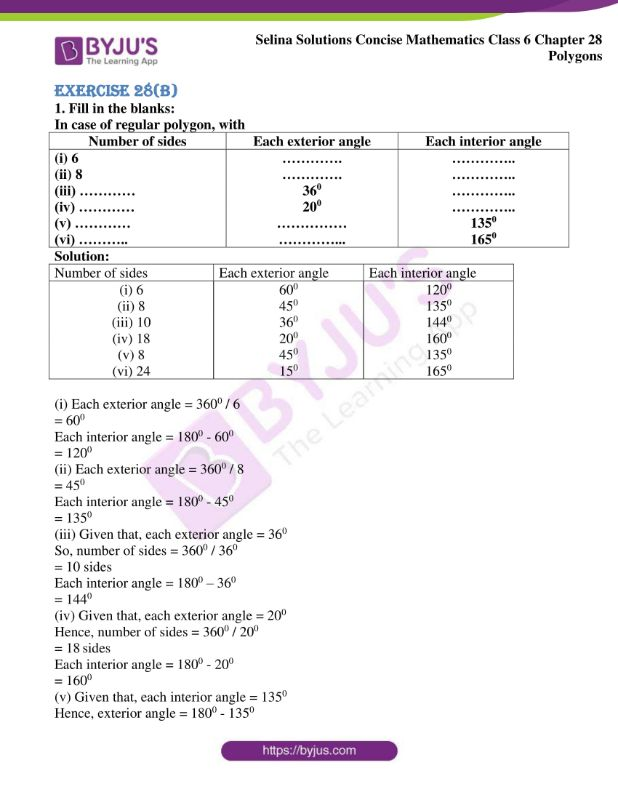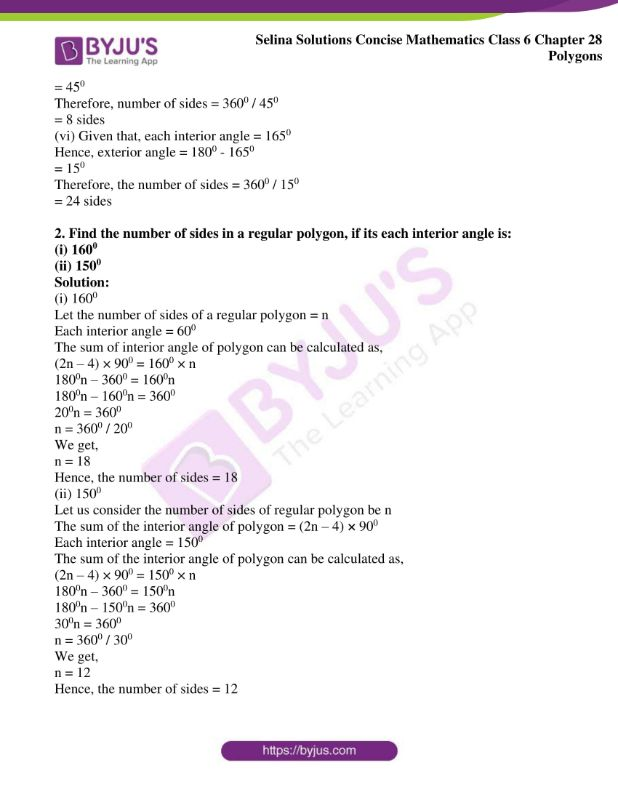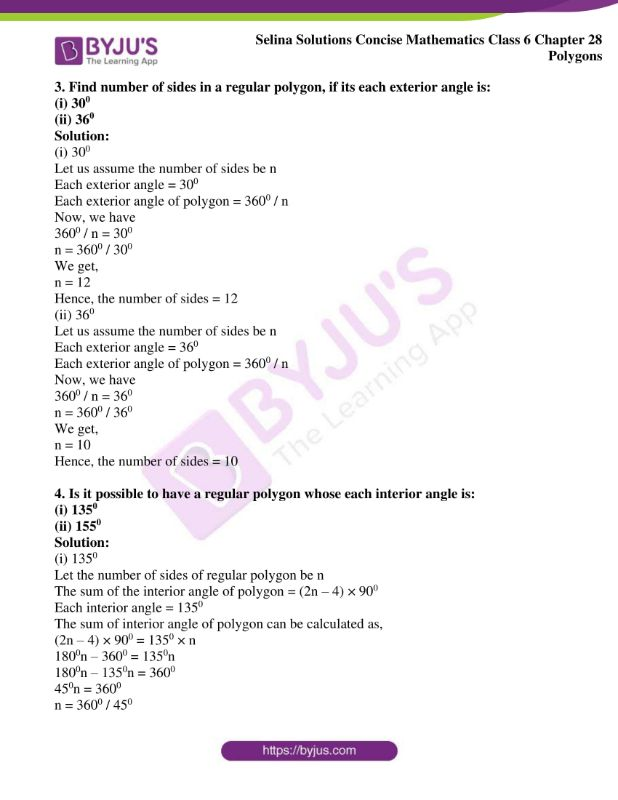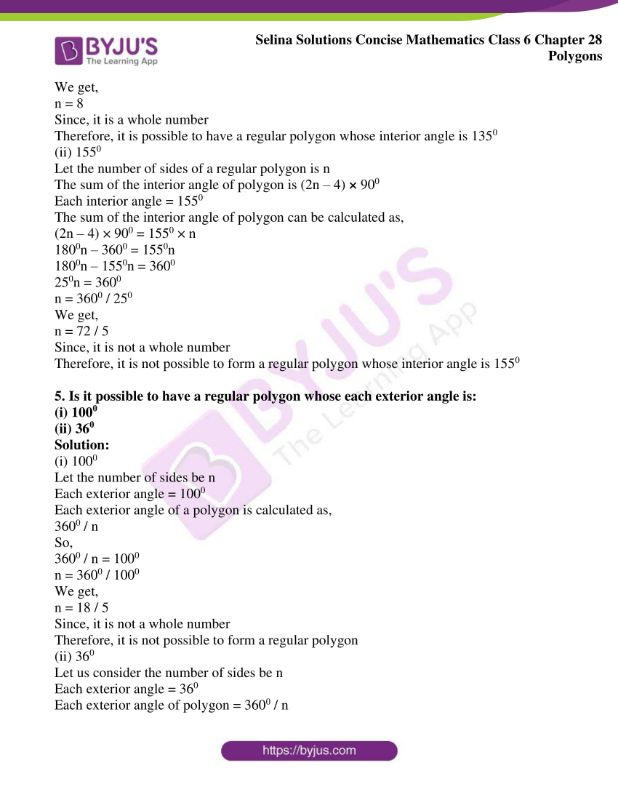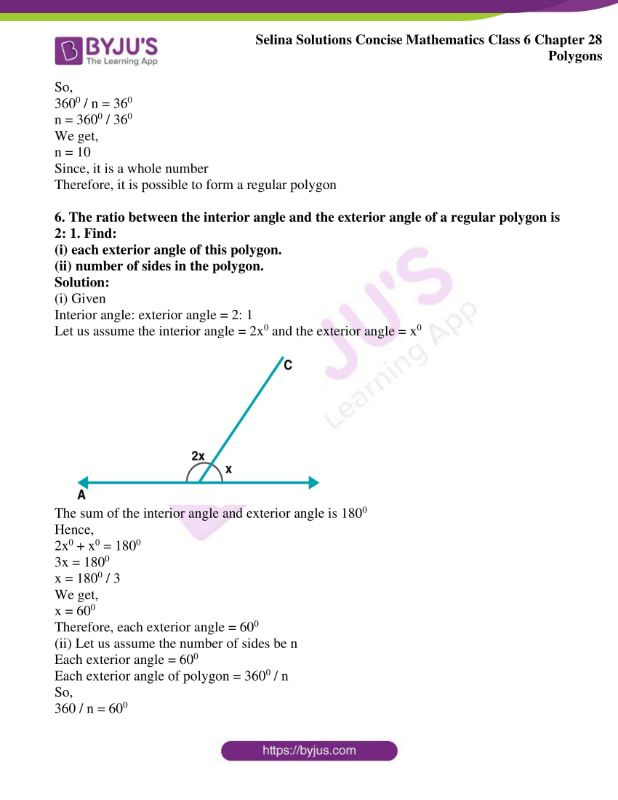### Access another exercise of Selina Solutions Concise Mathematics Class 6 Chapter 28 Polygons

Exercise 28(A) Solutions

### Access Selina Solutions Concise Mathematics Class 6 Chapter 28 Polygons Exercise 28(B)

Exercise 28(B)

1. Fill in the blanks:

In case of regular polygon, with

 Number of sides Each exterior angle Each interior angle (i) 6 …………. ………….. (ii) 8 …………. ………….. (iii) ………… 360 ………….. (iv) ………… 200 ………….. (v) ………… …………… 1350 (vi) ……….. …………… 1650

Solution:

 Number of sides Each exterior angle Each interior angle (i) 6 600 1200 (ii) 8 450 1350 (iii) 10 360 1440 (iv) 18 200 1600 (v) 8 450 1350 (vi) 24 150 1650

(i) Each exterior angle = 3600 / 6

= 600

Each interior angle = 1800 – 600

= 1200

(ii) Each exterior angle = 3600 / 8

= 450

Each interior angle = 1800 – 450

= 1350

(iii) Given that, each exterior angle = 360

So, number of sides = 3600 / 360

= 10 sides

Each interior angle = 1800 – 360

= 1440

(iv) Given that, each exterior angle = 200

Hence, number of sides = 3600 / 200

= 18 sides

Each interior angle = 1800 – 200

= 1600

(v) Given that, each interior angle = 1350

Hence, exterior angle = 1800 – 1350

= 450

Therefore, number of sides = 3600 / 450

= 8 sides

(vi) Given that, each interior angle = 1650

Hence, exterior angle = 1800 – 1650

= 150

Therefore, the number of sides = 3600 / 150

= 24 sides

2. Find the number of sides in a regular polygon, if its each interior angle is:

(i) 1600

(ii) 1500

Solution:

(i) 1600

Let the number of sides of a regular polygon = n

Each interior angle = 600

The sum of interior angle of polygon can be calculated as,

(2n – 4) × 900 = 1600 × n

1800n – 3600 = 1600n

1800n – 1600n = 3600

200n = 3600

n = 3600 / 200

We get,

n = 18

Hence, the number of sides = 18

(ii) 1500

Let us consider the number of sides of regular polygon be n

The sum of the interior angle of polygon = (2n – 4) × 900

Each interior angle = 1500

The sum of the interior angle of polygon can be calculated as,

(2n – 4) × 900 = 1500 × n

1800n – 3600 = 1500n

1800n – 1500n = 3600

300n = 3600

n = 3600 / 300

We get,

n = 12

Hence, the number of sides = 12

3. Find number of sides in a regular polygon, if its each exterior angle is:

(i) 300

(ii) 360

Solution:

(i) 300

Let us assume the number of sides be n

Each exterior angle = 300

Each exterior angle of polygon = 3600 / n

Now, we have

3600 / n = 300

n = 3600 / 300

We get,

n = 12

Hence, the number of sides = 12

(ii) 360

Let us assume the number of sides be n

Each exterior angle = 360

Each exterior angle of polygon = 3600 / n

Now, we have

3600 / n = 360

n = 3600 / 360

We get,

n = 10

Hence, the number of sides = 10

4. Is it possible to have a regular polygon whose each interior angle is:

(i) 1350

(ii) 1550

Solution:

(i) 1350

Let the number of sides of regular polygon be n

The sum of the interior angle of polygon = (2n – 4) × 900

Each interior angle = 1350

The sum of interior angle of polygon can be calculated as,

(2n – 4) × 900 = 1350 × n

1800n – 3600 = 1350n

1800n – 1350n = 3600

450n = 3600

n = 3600 / 450

We get,

n = 8

Since, it is a whole number

Therefore, it is possible to have a regular polygon whose interior angle is 1350

(ii) 1550

Let the number of sides of a regular polygon is n

The sum of the interior angle of polygon is (2n – 4) × 900

Each interior angle = 1550

The sum of the interior angle of polygon can be calculated as,

(2n – 4) × 900 = 1550 × n

1800n – 3600 = 1550n

1800n – 1550n = 3600

250n = 3600

n = 3600 / 250

We get,

n = 72 / 5

Since, it is not a whole number

Therefore, it is not possible to form a regular polygon whose interior angle is 1550

5. Is it possible to have a regular polygon whose each exterior angle is:

(i) 1000

(ii) 360

Solution:

(i) 1000

Let the number of sides be n

Each exterior angle = 1000

Each exterior angle of a polygon is calculated as,

3600 / n

So,

3600 / n = 1000

n = 3600 / 1000

We get,

n = 18 / 5

Since, it is not a whole number

Therefore, it is not possible to form a regular polygon

(ii) 360

Let us consider the number of sides be n

Each exterior angle = 360

Each exterior angle of polygon = 3600 / n

So,

3600 / n = 360

n = 3600 / 360

We get,

n = 10

Since, it is a whole number

Therefore, it is possible to form a regular polygon

6. The ratio between the interior angle and the exterior angle of a regular polygon is 2: 1. Find:

(i) each exterior angle of this polygon.

(ii) number of sides in the polygon.

Solution:

(i) Given

Interior angle: exterior angle = 2: 1

Let us assume the interior angle = 2x0 and the exterior angle = x0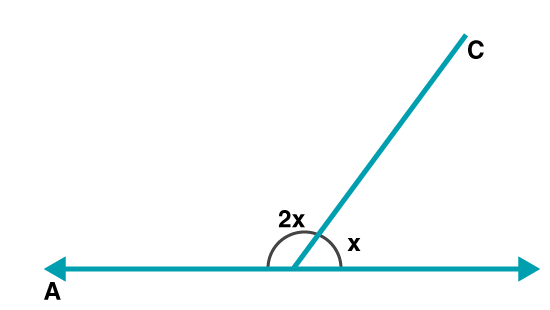The sum of the interior angle and exterior angle is 1800

Hence,

2x0 + x0 = 1800

3x = 1800

x = 1800 / 3

We get,

x = 600

Therefore, each exterior angle = 600

(ii) Let us assume the number of sides be n

Each exterior angle = 600

Each exterior angle of polygon = 3600 / n

So,

360 / n = 600

n = 3600 / 600

We get,

n = 6

Hence, the number of sides = 6